# deepSignalAnomalyDetectorCNN

Detect signal anomalies using 1-D convolutional autoencoder

Since R2023a

## Description

The `deepSignalAnomalyDetectorCNN` object uses a 1-D convolutional autoencoder model to detect signal anomalies.

## Creation

Create a `deepSignalAnomalyDetectorCNN` object using `deepSignalAnomalyDetector` and specifying `"conv"` as the model type.

## Properties

expand all

### General

Training status, specified as `0` (`false`) or `1` (`true`). When the detector has been trained, `IsTrained` is equal to `1`.

Data Types: `logical`

Number of channels in each input signal, specified as a positive integer.

Data Types: `single` | `double` | `int8` | `int16` | `int32` | `int64` | `uint8` | `uint16` | `uint32` | `uint64`

### Model

Type of deep learning model implemented by the detector, specified as `"conv"`.

Number of convolutional layers in the downsampling section of the model, specified as a positive integer.

The model uses the same number of transposed convolutional layers in the upsampling section, with the parameters in reverse order.

Data Types: `single` | `double` | `int8` | `int16` | `int32` | `int64` | `uint8` | `uint16` | `uint32` | `uint64`

Downsampling factor, specified as a positive integer or a vector of positive integers. When `DownsampleFactor` is a scalar, each layer uses the same downsampling factor. When `DownsampleFactor` is a vector, the ith layer uses a downsampling factor equal to the value of the ith element in the vector.

Data Types: `single` | `double` | `int8` | `int16` | `int32` | `int64` | `uint8` | `uint16` | `uint32` | `uint64`

Number of filters in downsampling convolution layers, specified as a positive scalar integer or a vector of positive integers.

Data Types: `single` | `double` | `int8` | `int16` | `int32` | `int64` | `uint8` | `uint16` | `uint32` | `uint64`

Size of filters in downsampling convolution layers, specified as a positive scalar integer or a vector of positive integers.

Data Types: `single` | `double` | `int8` | `int16` | `int32` | `int64` | `uint8` | `uint16` | `uint32` | `uint64`

Dropout probability used to avoid overfitting, specified as a nonnegative scalar less than 1.

Data Types: `single` | `double` | `int8` | `int16` | `int32` | `int64` | `uint8` | `uint16` | `uint32` | `uint64`

### Window

Window length of each signal segment, specified as a positive integer or as `"fullSignal"`.

• If `WindowLength` is specified as an integer, the detector divides each input signal into segments. The length of each segment is equal to the specified value in samples.

• If `WindowLength` is specified as `"fullSignal"`, the detector treats each input signal as a single segment.

Use `updateDetector` to modify this and other window properties.

Data Types: `single` | `double` | `int8` | `int16` | `int32` | `int64` | `uint8` | `uint16` | `uint32` | `uint64` | `char` | `string`

Number of overlapped samples between window segments, specified as a positive integer or as `"auto"`.

• If `OverlapLength` is specified as integer, the detector sets the number of overlapped samples equal to the specified value.

• If `OverlapLength` is specified as `"auto"`, the detector sets the number of overlapped samples equal to `WindowLength` – 1.

Data Types: `single` | `double` | `int8` | `int16` | `int32` | `int64` | `uint8` | `uint16` | `uint32` | `uint64` | `char` | `string`

Method to aggregate sample loss within each window segment, specified as one of these:

• `"max"` — Compute the aggregated window loss as the maximum value of all the sample losses within the window.

• `"mean"` — Compute the aggregated window loss as the mean value of all the sample losses within the window.

• `"median"` — Compute the aggregated window loss as the median value of all the sample losses within the window.

• `"min"` — Compute the aggregated window loss as the minimum value of all the sample losses within the window.

Data Types: `char` | `string`

### Threshold

Method to compute the detection threshold, specified as one of these:

• `"contaminationFraction"` — Value corresponding to the detection of anomalies within a specified fraction of windows. The fraction value is specified by `ThresholdParameter`.

• `"max"` — Maximum window loss measured over the entire training data set and multiplied by `ThresholdParameter`.

• `"median"` — Median window loss measured over the entire training data set and multiplied by `ThresholdParameter`.

• `"mean"` — Mean window loss measured over the entire training data set and multiplied by `ThresholdParameter`.

• `"manual"` — Manual detection threshold value based on `Threshold`.

• `"customFunction"` — Custom detection threshold value based on `ThresholdFunction`.

Use `updateDetector` to modify this and other threshold properties.

Data Types: `char` | `string`

Detection threshold, specified as a nonnegative scalar when `ThresholdMethod` is set to `"contaminationFraction"`, and as positive scalar when `ThresholdMethod` is set to `"max"`, `"median"`, or `"mean"`.

Data Types: `single` | `double` | `int8` | `int16` | `int32` | `int64` | `uint8` | `uint16` | `uint32` | `uint64`

Manual detection threshold, specified as a positive scalar. This property applies only when `ThresholdMethod` is set to `"manual"`.

Data Types: `single` | `double` | `int8` | `int16` | `int32` | `int64` | `uint8` | `uint16` | `uint32` | `uint64`

Function to compute custom detection threshold, specified as a function handle. This property applies only when `ThresholdMethod` is set to `"customFunction"`.

Data Types: `function_handle`

## Object Functions

 `detect` Detect anomalies in signals `getModel` Get underlying neural network model of signal anomaly detector `plotAnomalies` Plot signal anomalies `plotLoss` Plot window reconstruction loss `plotLossDistribution` Plot CDF and histogram of aggregated window loss distribution `trainDetector` Train signal anomaly detector `updateDetector` Update settings of trained detector and recompute detection threshold

## Examples

collapse all

Load the file `sineWaveAnomalyData.mat`, which contains two sets of synthetic three-channel sinusoidal signals.

`sineWaveNormal` contains 10 sinusoids of stable frequency and amplitude. Each signal has a series of small-amplitude impact-like imperfections. The signals have different lengths and initial phases. Plot the first three normal signals.

```load sineWaveAnomalyData.mat sl = 3; tiledlayout("vertical") ax = zeros(sl,1); for kj = 1:sl ax(kj) = nexttile; plot(sineWaveNormal{kj}) title("Normal Signal") end```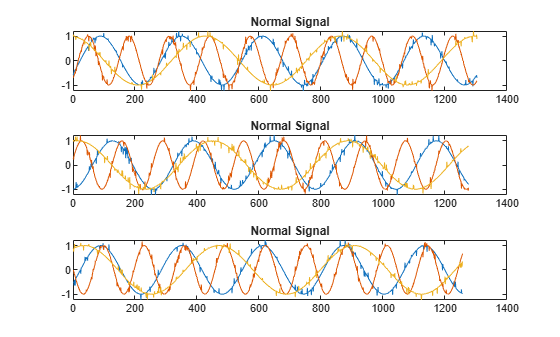`sineWaveAbnormal` contains three signals, all of the same length. Each signal in the set has one or more anomalies.

• All channels of the first signal have an abrupt change in frequency that lasts for a finite time.

• The second signal has a finite-duration amplitude change in one of its channels.

• The third signal has spikes at random times in all channels.

Plot the three signals with anomalies.

```for kj = 1:sl plot(ax(kj),sineWaveAbnormal{kj}) title(ax(kj),"Signal with Anomalies") end```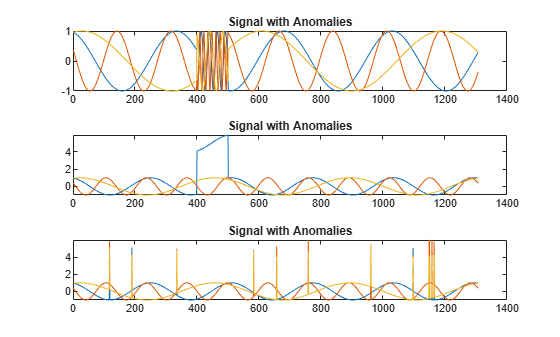Create an autoencoder object to detect the anomalies in the abnormal signals. The object contains a neural network that you can train to best reproduce an input set of anomaly-free data. The trained object encodes the patterns inherent in the data and can recognize if a signal contains regions that deviate from the patterns. You do not need to label any data to train the detector. The training process is unsupervised.

By default, the `deepSignalAnomalyDetector` function creates objects with convolutional autoencoders. The only mandatory argument is the number of channels in the training and testing signals.

`D = deepSignalAnomalyDetector(sl)`
```D = deepSignalAnomalyDetectorCNN with properties: IsTrained: 0 NumChannels: 3 Model Information ModelType: 'conv' FilterSize: 8 NumFilters: 32 NumDownsampleLayers: 2 DownsampleFactor: 2 DropoutProbability: 0.2000 Threshold Information Threshold: [] ThresholdMethod: 'contaminationFraction' ThresholdParameter: 0.0100 Window Information WindowLength: 1 OverlapLength: 'auto' WindowLossAggregation: 'mean' ```

Use the `trainDetector` function to train the convolutional neural network with the normal data. Use the training options for the adaptive moment estimation (Adam) optimizer. Specify a maximum number of 100 epochs to use for training. For more information, see `trainingOptions` (Deep Learning Toolbox).

```opts = trainingOptions("adam",MaxEpochs=100); trainDetector(D,sineWaveNormal,opts)```
```Training on single CPU. |========================================================================================| | Epoch | Iteration | Time Elapsed | Mini-batch | Mini-batch | Base Learning | | | | (hh:mm:ss) | RMSE | Loss | Rate | |========================================================================================| | 1 | 1 | 00:00:00 | 1.23 | 0.8 | 0.0010 | | 50 | 50 | 00:00:07 | 0.45 | 1.0e-01 | 0.0010 | | 100 | 100 | 00:00:15 | 0.38 | 7.2e-02 | 0.0010 | |========================================================================================| Training finished: Max epochs completed. Computing threshold... Threshold computation completed. ```

Use the trained detector to detect the anomalies in the abnormal data. The `detect` function outputs two cell arrays, with each array element corresponding to a signal in the testing data.

`[lbls,loss] = detect(D,sineWaveAbnormal);`

The first output of `detect` is a categorical array that declares each sample of a signal as being anomalous or not. A sample can be either a point, a signal region, or an entire signal. An anomaly is detected when the reconstruction loss, or the difference between the value of a signal and the value reconstructed by the detector based on the training data, exceeds a given threshold. You can set a threshold manually or you can direct the detector to compute a threshold based on a specified statistic of loss values computed on training data.

```for kj = 1:sl hold(ax(kj),"on") plot(ax(kj),lbls{kj},LineWidth=2) title(ax(kj),"Signal with Anomalies") hold(ax(kj),"off") end```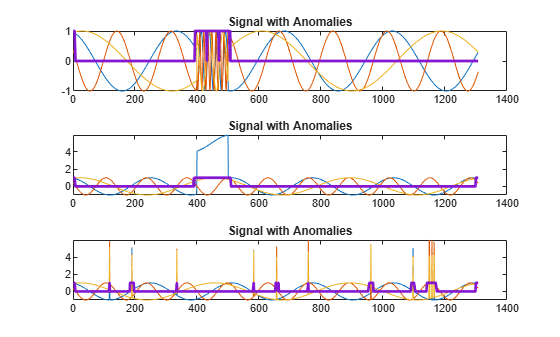Alternatively, use the `plotAnomalies` function to plot each channel of each signal and the anomalies found by the detector. The detector decides that there is an anomaly in a signal if it detects one in any of the signal channels. `plotAnomalies` plots the anomaly locations in all channels.

```for kj = 1:sl figure plotAnomalies(D,sineWaveAbnormal{kj}) end```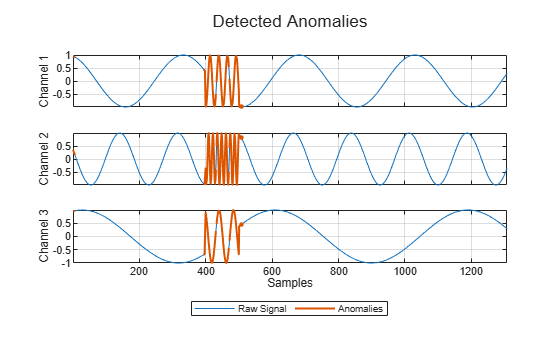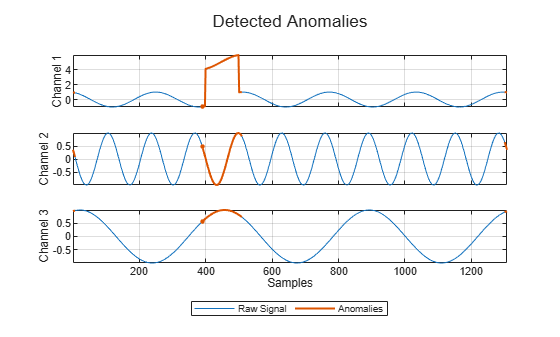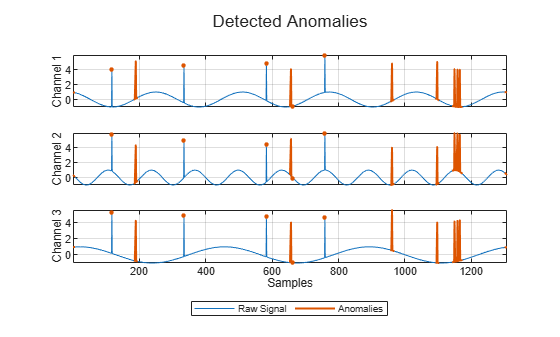The second output of `detect` is the computed reconstruction loss that the object uses to determine the labeling. You can plot the reconstruction loss directly from the output of `detect`, but it is more convenient to use the `plotLoss` function, which also displays the the threshold value above which a sample is declared to be anomalous.

```tiledlayout("vertical") for kj = 1:sl nexttile plotLoss(D,sineWaveAbnormal{kj}) end```The `plotLossDistribution` function displays the reconstruction loss distribution and gives insight into the way the detector chooses the threshold. You can plot the distribution loss for the normal data alongside the distribution loss for the abnormal data. The threshold chosen by the detector is larger than the loss values for most of the normal-data samples but smaller than an appreciable number of abnormal-data losses that signal the possible presence of anomalies. You can improve the choice of threshold by using the `updateDetector` function.

```clf plotLossDistribution(D,sineWaveNormal,sineWaveAbnormal)```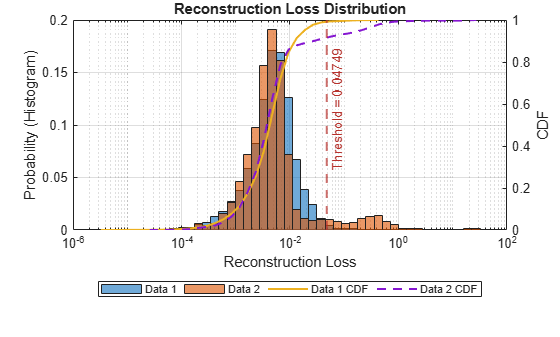Use the `getModel` function to obtain the underlying neural network model corresponding to the detector. You can then inspect the model using `analyzeNetwork` (Deep Learning Toolbox).

```M = getModel(D); analyzeNetwork(M)```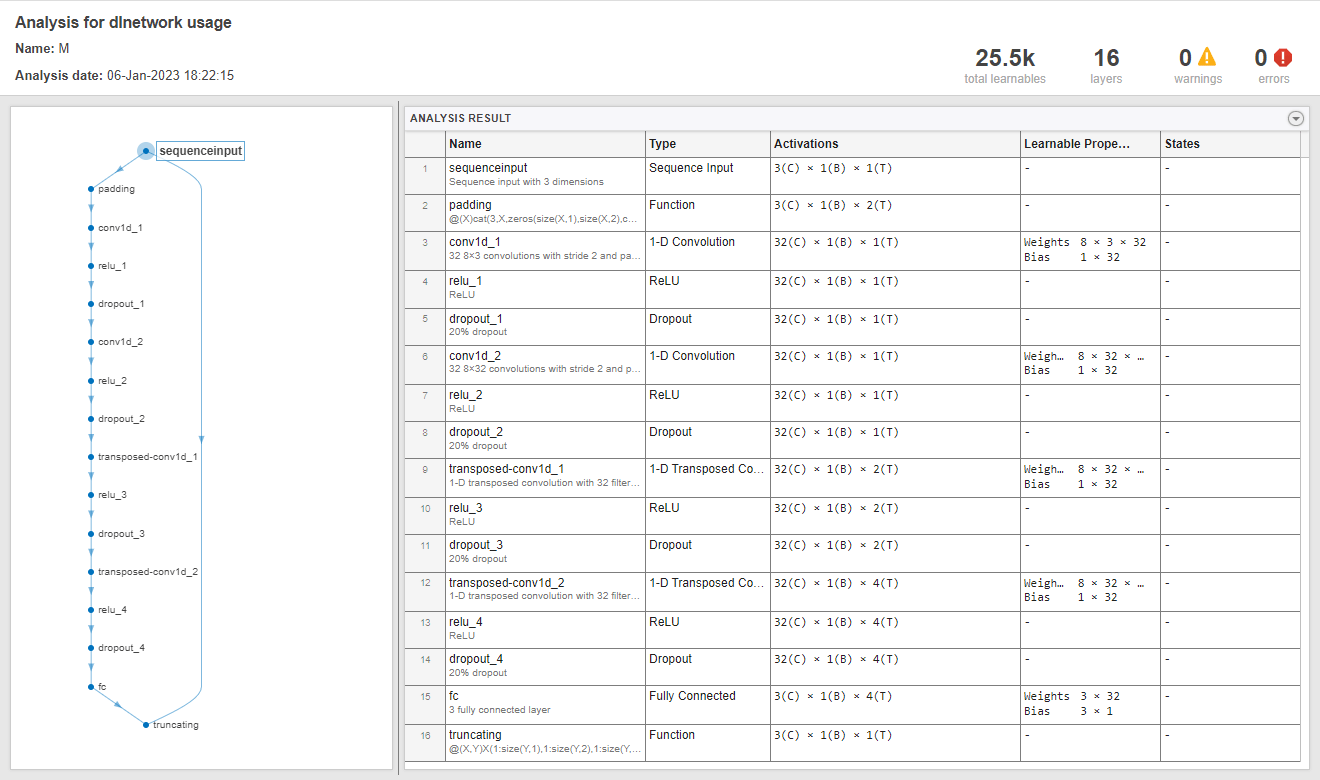## Version History

Introduced in R2023a